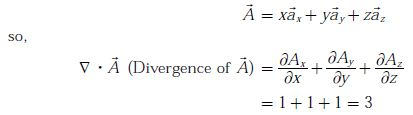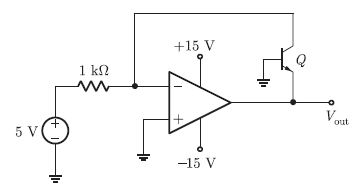# EC GATE 2013 - Online Test

Q1. Choose the grammatically CORRECT sentence:
Explaination / Solution:
No Explaination.

Q2. They were requested not to quarrel with others. Which one of the following options is the closest in meaning to the word quarrel?
Explaination / Solution:

They were requested not to quarrel with others. Quarrel has a similar meaning to ‘fall out’

Q3. Statement: You can always give me a ring whenever you need. Which one of the following is the best inference from the above statement?
Explaination / Solution:

You can always given me a ring whenever you need. Because a friend is need is a friend indeed

Q4. Statement: There were different streams of freedom movements in colonial India carried out by the moderates, liberals, radicals, socialists, and so on. Which one of the following is the best inference from the above statement?
Explaination / Solution:
No Explaination.

Q5. Complete the sentence: Dare .................. mistakes.
Explaination / Solution:
No Explaination.

Q6. The divergence of the vector fieldExplaination / Solution:

Given, the vector fieldQ7.
In the circuit shown below what is the output voltage  (vout)if a silicon transistor Q and an ideal op-amp are used?Explaination / Solution:

For the given ideal op-amp, negative terminal will be also ground (at zero voltage) and so, the collector terminal of the BJT will be at zero voltage.
i.e., VC = 0 volt
The current in 1 k resistor is given by
This current will flow completely through the BJT since, no current will flow into the ideal op-amp (I/P resistance of ideal op-amp is infinity). So, for BJT we havei.e.,the base collector junction is reverse biased (zero voltage) therefore, the collector current (IC ) can have a value only if base-emitter is forward biased.
Hence,Q8. In the circuit shown below the op-amps are ideal. Then, Vout in Volts isExplaination / Solution:
No Explaination.

Q9. In a forward biased pn junction diode, the sequence of events that best describes the mechanism of current flow is
Explaination / Solution:

The potential barrier of the pn junction is lowered when a forward bias voltage is applied, allowing electrons and holes to flow across the space charge region (Injection) when holes flow from the p region across the space charge region into the n region, they become excess minority carrier holes and are subject to diffuse, drift and recombination processes. Hence correct option is (A)

Q10. In IC technology, dry oxidation (using dry oxygen) as compared to wet oxidation (using steam or water vapor) produces
Explaination / Solution:

In IC technology, dry oxidation as compared to wet oxidation produces superior quality oxide with a lower growth rate